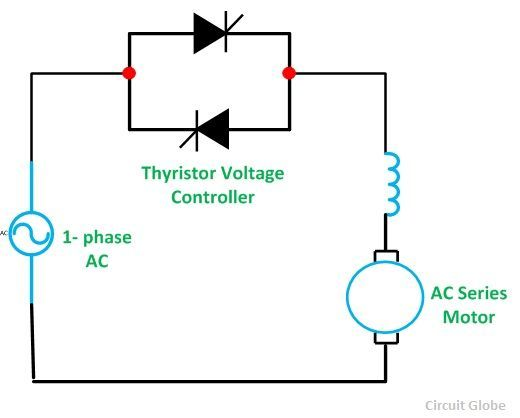# Stator Voltage Control of an Induction Motor

Stator Voltage Control is a method used to control the speed of an Induction Motor. The speed of a three-phase induction motor can be varied by varying the supply voltage. As we already know that the torque developed is proportional to the square of the supply voltage and the slip at the maximum torque is independent of the supply voltage. The variation in the supply voltage does not alter the synchronous speed of the motor.

The Torque-Speed Characteristics of the three-phase induction motors for varying supply voltage and also for fan load are shown below:By varying the supplying voltage, the speed can be controlled. The voltage is varied until the torque required by the load is developed, at the desired speed. The torque developed is proportional to the square of the supply voltage and the current is proportional to the voltage.

Hence, to reduce the speed for the same value of the same current, the value of the voltage is reduced and as a result, the torque developed by the motor is reduced. This stator voltage control method is suitable for applications where the load torque decreases with the speed. For example- In the fan load.

This method gives a speed control only below the normal rated speed as the operation of the voltages if higher than the rated voltage is not admissible. This method is suitable where the intermittent operation of the drive is required and also for the fan and pump drives. As in fan and pump the load torque varies as the square of the speed. These types of drives required low torque at lower speeds. This condition can be obtained by applying a lower voltage without exceeding the motor current.

The variable voltage for speed control of small size motors mainly for single-phase can be obtained by the following methods given below:

• By connecting an external resistance in the stator circuit of the motor.
• By using an Auto-transformer.
• By using a Thyristor voltage controller.
• By using a Triac Controller.

Nowadays the Thyristor voltage controller method is preferred for varying the voltage. For a single phase supply, two thyristors are connected back to back as shown in the figure below:The domestic fan motors, which are single-phase are controlled by a single-phase Triac Voltage Controller as shown in the figure below:Speed control is obtained by varying the firing angle of the Triac. These controllers are known as Solid State fan regulators. As the solid-state regulators are more compact and efficient as compared to the conventional variable regulator. Thus, they are preferred over the normal regulator.

In the case of a three-phase induction motor, three pairs of thyristors are required which are connected back to back. Each pair consists of two thyristors. The diagram below shows the Stator Voltage Control of the three-phase induction motors by Thyristor Voltage Controller.Each pair of the thyristor controls the voltage of the phase to which it is connected. Speed control is obtained by varying the conduction period of the Thyristor. For lower power ratings, the back-to-back thyristor pairs connected in each phase are replaced by the Traic.